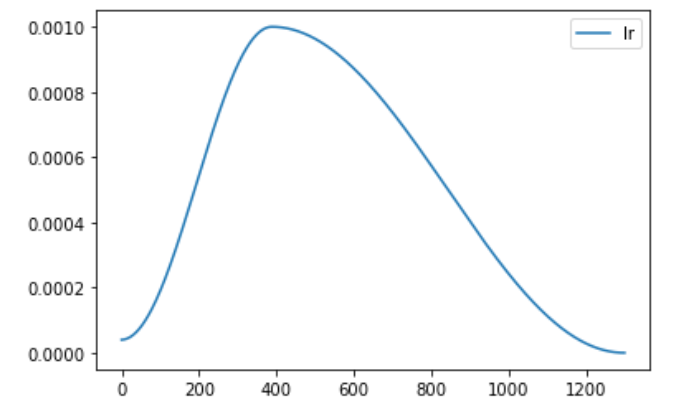# `optimizer.step()` before `lr_scheduler.step()` error using GradScaler

Even though I think my code calls the `optimizer.step` via `Gradscaler` function before the `lr_scheduler.step()` function I am still getting this warning:

/opt/anaconda3/envs/huggingface/lib/python3.7/site-packages/torch/optim/lr_scheduler.py:123: UserWarning: Detected call of `lr_scheduler.step()` before `optimizer.step()`. In PyTorch 1.1.0 and later, you should call them in the opposite order: `optimizer.step()` before `lr_scheduler.step()`. Failure to do this will result in PyTorch skipping the first value of the learning rate schedule.

As you can see in my training code `scaler.step(optimizer)` gets called before `scheduler.step()`, but I am still getting this warning. Any ideas what might be wrong?

Here is my training code.

``````    steps = len(train_dl) * epochs
scheduler = torch.optim.lr_scheduler.OneCycleLR(optimizer, max_lr=lr, steps_per_epoch=len(train_dl), epochs=epochs)
avg_train_losses = []
avg_val_losses = []
avg_val_scores = []
lr = []
best_avg_val_score = -1000
scaler = torch.cuda.amp.GradScaler() # mixed precision support
for epoch in tqdm(range(epochs), total=epochs):
model.train()
total_train_loss = 0.0
for i, (x, y, image_tensor) in enumerate(train_dl):
x, y, image_tensor = move_to_dev(x, y, image_tensor)
with torch.cuda.amp.autocast():
output = model(x, image_tensor)
loss = criterion(y, output)
total_train_loss += loss.item()

# Backward Pass and Optimization
scaler.scale(loss).backward()
scaler.step(optimizer)
scaler.update()
scheduler.step()
lr.append(get_lr(optimizer))
``````
2 Likes

If the first iteration creates NaN gradients (e.g. due to a high scaling factor and thus gradient overflow), the `optimizer.step()` will be skipped and you might get this warning.

You could check the scaling factor via `scaler.get_scale()` and skip the learning rate scheduler, if it was decreased. I think it might be useful to add a utility function (or return value in `scaler.step()`) to indicate, if the current `optimizer.step()` was skipped.

4 Likes

@ptrblck many thanks for your answer, really appreciate you took time for this. I am using the `torch.optim.lr_scheduler.OneCycleLR` scheduler (`torch.optim.lr_scheduler.OneCycleLR(optimizer, max_lr=1e-3, steps_per_epoch=len(train_dl), epochs=epochs)`) and at the beginning of the training the learning rate might be very small. The actual learning rate curve looks like this:So could it be that because the learning rate is very small at the first iteration, that this is causing this `NaN` gradients?

1 Like

A small learning rate should reduce the probability of overflows in the gradients, which could create NaNs. However, if the scale factor is still too high, even a low learning rate might cause it.
Could you check, if the scale factor was reduced in the first iteration(s)?

2 Likes

@ptrblck Just checked the documentation of the `GradScaler` class and found this:

The scale factor often causes infs/NaNs to appear in gradients for the first few iterations as its value calibrates. `scaler.step` will skip the underlying `optimizer.step()` for these iterations. After that, step skipping should occur rarely (once every few hundred or thousand iterations).

Could this be the cause for such warnings?

And another question: do you get the scale factor using `scaler.get_scale()` where `scaler` is an instance of `torch.cuda.amp.GradScaler`?

Yes, exactly. That’s what I was trying to say here:

Sorry, if I couldn’t describe it clearly.Yes, that’s what I had in mind.

I have tried to capture the scale factor on each batch (100 epochs, batch size: 64, 24 batches per epoch) and then plot it:Right now I am not getting the warning, but still it shows up some now and then. Anyway I think that we know what is causing this issue. @ptrblck Many thanks for your pointers.

1 Like

Thank you all very much for the help. It wasn’t that clear but I could figure it out. The way I did it:

``````        ...

scale = scaler.get_scale()
scaler.update()
skip_lr_sched = (scale != scaler.get_scale())
...

if not skip_lr_sched:
lr_sched.step()
...``````
3 Likes

So this solution checks that the scale is NONE rather than just really large? Does this work, since I am encountering the same thing.

I know this is an old post, but just wanted to get this out there in case it helps someone.

I would suggest checking:
`skip_lr_sched = (scale > scaler.get_scale())`
`skip_lr_sched = (scale != scaler.get_scale())`
because according to the docs, `scaler.update()` decreases the `scale_factor` when `optimizer.step()` is skipped, as well as increases the `scale_factor` when `optimizer.step()` has not been skipped for `growth_interval` consecutive iterations.
Simply checking `scale != scaler.get_scale()` will return `False` even when the `scale_factor` is increased (and `optimizer.step` has NOT been skipped), which we don’t want.